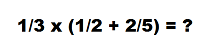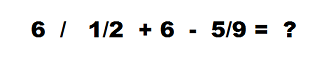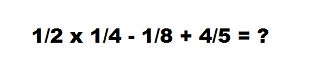# Order of Operations with Fractions

Instructions:

question 1 of 3

### What is the first thing you should do to solve this equation?Create Your Account To Take This Quiz

As a member, you'll also get unlimited access to over 79,000 lessons in math, English, science, history, and more. Plus, get practice tests, quizzes, and personalized coaching to help you succeed.

Try it risk-free for 30 days. Cancel anytime

### 1. What is the first thing you should do to solve this equation?### 2. What is the correct order of operations for this equation?Create your account to access this entire worksheet
Quizzes, practice exams & worksheets
Certificate of Completion
Create an account to get started

This quiz and worksheet is an assessment to check what you know about solving math problems with fractions. On the quiz, you'll be asked how to decide the order of operations, and you'll need to solve sample problems.

## Quiz & Worksheet Goals

The purpose of this quiz and worksheet is to test what you know about:

• The first step to adding fractions together
• Identifying the operations in an equation with fractions
• Defining the order of operations
• Solving problems with fractions and multiple operations

## Skills Practiced

• Information recall - see if you remember the key step to adding fractions with different denominators
• Knowledge application - review what you know about working with fractions to answer questions about the order of operations
• Problem solving - use what you know of math problems involving fractions to solve practice problems for the correct answer

You'll find a deeper review of this topic in the lesson titled Order of Operations with Fractions. This lesson provides more insight into:

• The importance of the order of operations
• The mnemonic device used to remember the order of operations
• Sample problems to review
Final ExamMath for Kids
Status: Not Started
Chapter ExamFraction Operations for Elementary School
Status: Not Started

Support